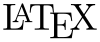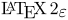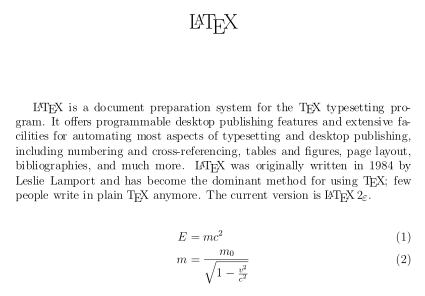# LaTeX facts for kids

Kids Encyclopedia Facts
Latex is also the sap of certain trees that can be used to make rubber
Original author(s)Leslie Lamport Cross-platform Typesetting LaTeX Project Public License (LPPL)

LaTeX is a computer program used for making articles, books and mathematical formulas more aesthetically pleasing. LatTex is well-suited for expressing mathematical formulas on electronic devices in a more human readable format by allowing them to be shown in a format similar to how they would be written in many textbooks or by hand. LaTeX is used for making mathematical formulas for some articles on Wikipedia in addition to being used within academic circles.

The writer types his article into a plain text document. A plain text document cannot have styled text, like bold or italic. When the writer wants to write styled text, they use Computer Commands. For example, the command for bold text is \textbf{This text is bold}. After the writer is finished writing the article, they tell LaTeX to read the document. After LaTeX is done, LaTeX makes a file that can be printed. The command \textbf{This text is bold} would print as This text is bold.

LaTeX was first made in the early 1980s by Leslie Lamport at SRI International. The current version is LaTeX2e (styled).

## Example

The example below shows the LaTeX input and corresponding output:

 \documentclass[12pt]{article} \usepackage{amsmath} \title{\LaTeX} \date{} \begin{document} \maketitle \LaTeX{} is a document preparation system for the \TeX{} typesetting program. It offers programmable desktop publishing features and extensive facilities for automating most aspects of typesetting and desktop publishing, including numbering and cross-referencing, tables and figures, page layout, bibliographies, and much more. \LaTeX{} was originally written in 1984 by Leslie Lamport and has become the dominant method for using \TeX; few people write in plain \TeX{} anymore. The current version is \LaTeXe. % This is a comment; it will not be shown in the final output. % The following shows a little of the typesetting power of LaTeX: \begin{align} E &= mc^2 \\ m &= \frac{m_0}{\sqrt{1-\frac{v^2}{c^2}}} \end{align} \end{document}LaTeX Facts for Kids. Kiddle Encyclopedia.